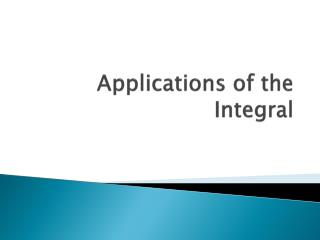DownloadDownload PresentationApplications of the Integral

# Applications of the Integral

Download Presentation## Applications of the Integral

- - - - - - - - - - - - - - - - - - - - - - - - - - - E N D - - - - - - - - - - - - - - - - - - - - - - - - - - -
##### Presentation Transcript

1. Applications of the Integral

2. Areas

3. Example (1)Find the area A of the region bounded by:y=x2 +1, y=x , x=0 & x=1.

4. Intersections of the Two Curves

5. Example (3)Find the area A of the region bounded by:y=cosx, y=sinx, x=0 and x=π/2

6. Intersection of the Two Graphs

7. Example (4)Find the area A of the region in the first quadranbounded by:y=x2+ 1 and y-10=0 We second curve’s equation can be rewritten as: y = 10 The curves intersects at the points x=3 and x=-3. We arrive at that by letting x2+ 1=10, which leads to x2=9 The following figures shows the mentioned region. Find the area using two methods!

8. Example (5)Set up the integral/integrals to find the area A of the region bounded by:y=9-x2, y=x2 + 1 from x=-4 to x=3